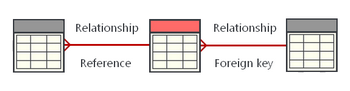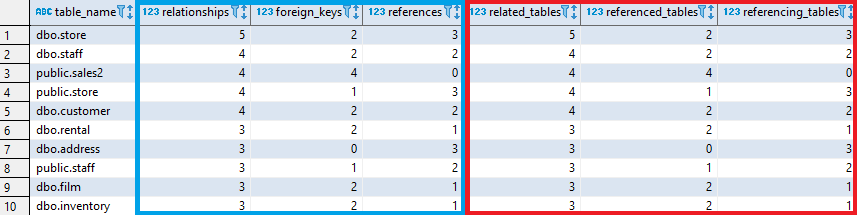## List tables with most relationships in Redshift

Article for:

Query below lists tables with most relationships (both foreign keys and FK references from other tables).## Query

``````select relations.table_name as table_name,
count(relations.table_name) as relationships,
count(relations.referenced_tables) as foreign_keys,
count(relations.referencing_tables) as references,
count(relations.related_table) as related_tables,
count(relations.referenced_tables) as referenced_tables,
count(relations.referencing_tables) as referencing_tables
from(
select pk_tco.table_schema || '.' || pk_tco.table_name as table_name,
(fk_tco.table_schema || '.' || fk_tco.table_name) as related_table,
fk_tco.table_name as referencing_tables,
null as referenced_tables
from information_schema.referential_constraints rco
join information_schema.table_constraints fk_tco
on rco.constraint_name = fk_tco.constraint_name
and rco.constraint_schema = fk_tco.table_schema
join information_schema.table_constraints pk_tco
on rco.unique_constraint_name = pk_tco.constraint_name
and rco.unique_constraint_schema = pk_tco.table_schema
union all
select fk_tco.table_schema || '.' || fk_tco.table_name as table_name,
pk_tco.table_schema || '.' || pk_tco.table_name as related_table,
null as referencing_tables,
pk_tco.table_name as referenced_tables
from information_schema.referential_constraints rco
join information_schema.table_constraints fk_tco
on rco.constraint_name = fk_tco.constraint_name
and rco.constraint_schema = fk_tco.table_schema
join information_schema.table_constraints pk_tco
on rco.unique_constraint_name = pk_tco.constraint_name
and rco.unique_constraint_schema = pk_tco.table_schema
) relations
group by table_name
order by relationships desc;
``````

## Columns

• table_name - table name with schema name
• relationships - number of table relationships
• foreign_keys - number of foreign keys in a table
• references - number of foreign keys that are refering to this table
• related_tables - number of different related tables (regardless of relationship type/direction table is counted only once)
• referenced_tables - number of different tables referenced with foreign key
• referencing_tables - nubmer of different tables referencing with foreign keys this table

Note: Multiple FK's can refers to one table, so number of foreign keys might be diffrent than number of referenced tables (same property applies to references and referencing tables)

## Rows

• One row represents one table
• Scope of rows: all tables with any relationship
• Ordered by relationships descending

## Sample results